Tools, Technologies and Training for Healthcare Laboratories

# Normalized OPSpecs Chart N=4 90% AQA

This is a Normalized OPSpecs chart 90% AQA (analytical quality assurance) and with N=4 (4 control measurements).

# QC Selection Grid, Square 1

This is a discussion of part of the QC Selection Grid.

# QC Selection Grid, Square 2

## MULTIRULE CHOICE: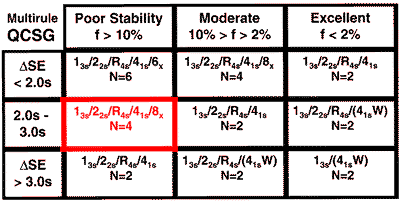This is not a good situation because you have frequent problems, however, medically important errors are moderate in size and may be detectable by using a five rule procedure with N=4. The 8x rule should be used across runs and interpreted as a rejection rule. The power curves below show the rejection characteristics within a run (R=1) and across two runs (R=2):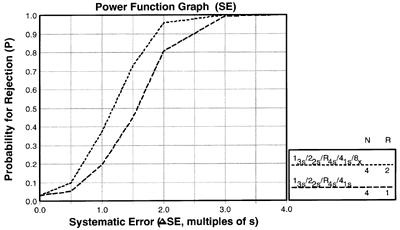Plot your critical-error on the x-axis of this power function graph. Draw a vertical line from that point to intersect the power curves. Where the curves and critical-error meet indicates how much error detection each control rule will provide.

# QC Selection Grid, Square 3

## MULTIRULE CHOICE: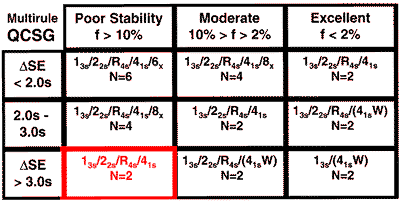You have many problems to detect, but fortunately medically important errors are large in size and may be detectable. It is recommended that you increase the number of rules to four and apply the 41s rule across two runs (R=2) as a rejection rule. The power curves below show the rejection characteristics within one run (R=1) and across two runs (R=2):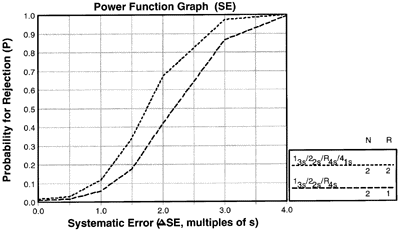Plot your critical-error on the x-axis of this power function graph. Draw a vertical line from that point to intersect the power curves. Where the curves and critical-error meet indicates how much error detection each control rule will provide.

# QC Selection Grid, Square 4

## MULTIRULE CHOICE: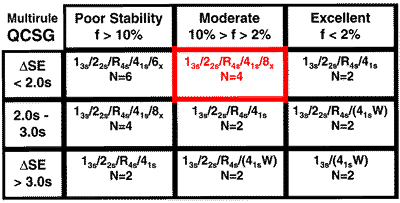This is not a good situation because you have a moderate number of problems and medically important errors are small and difficult to detect. You should use a five rule procedure with N=4. The 8x rule should be used across runs and interpreted as a rejection rule. The power curves below show the rejection characteristics within a run (R=1) and across two runs (R=2):Plot your critical-error on the x-axis of this power function graph. Draw a vertical line from that point to intersect the power curves. Where the curves and critical-error meet indicates how much error detection each control rule will provide.

### More Articles ...

Joomla SEF URLs by Artio